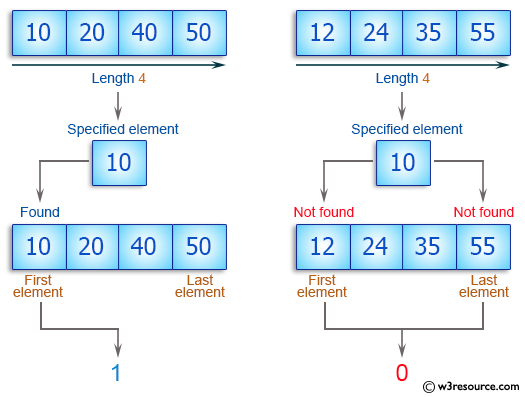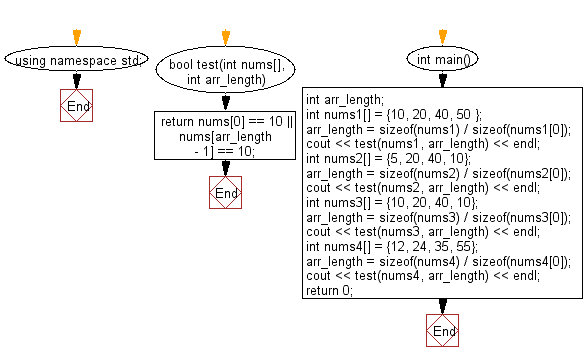﻿ C++ : Check 10 appears as either the first, last in an array# C++ Exercises: Check a given array of integers of length 1 or more and return true if 10 appears as either first or last element in the given array

## C++ Basic Algorithm: Exercise-81 with Solution

Write a C++ program to check a given array of integers of length 1 or more and return true if 10 appears as either first or last element in the given array.

Sample Solution:

C++ Code :

``````#include <iostream>
using namespace std;

bool test(int nums[], int arr_length)
{
return nums == 10 || nums[arr_length - 1] == 10;
}

int main()
{
int arr_length;
int nums1[] = {10, 20, 40, 50 };
arr_length = sizeof(nums1) / sizeof(nums1);
cout << test(nums1, arr_length) << endl;
int nums2[] = {5, 20, 40, 10};
arr_length = sizeof(nums2) / sizeof(nums2);
cout << test(nums2, arr_length) << endl;
int nums3[] = {10, 20, 40, 10};
arr_length = sizeof(nums3) / sizeof(nums3);
cout << test(nums3, arr_length) << endl;
int nums4[] = {12, 24, 35, 55};
arr_length = sizeof(nums4) / sizeof(nums4);
cout << test(nums4, arr_length) << endl;

return 0;
}
``````

Sample Output:

```1
1
1
0
```

Pictorial Presentation:Flowchart:C++ Code Editor:

Contribute your code and comments through Disqus.

What is the difficulty level of this exercise?

﻿